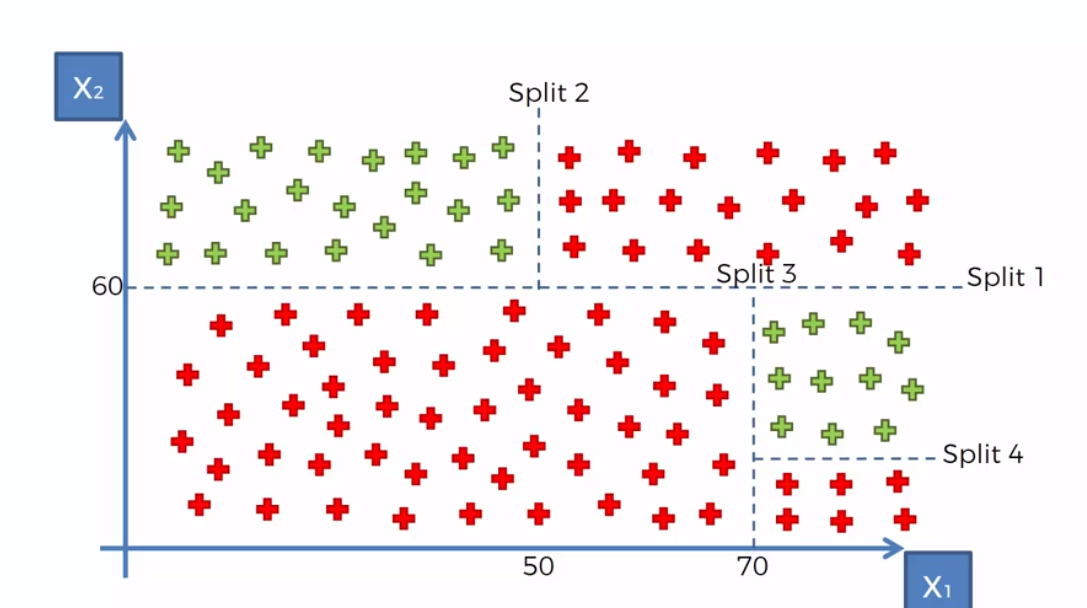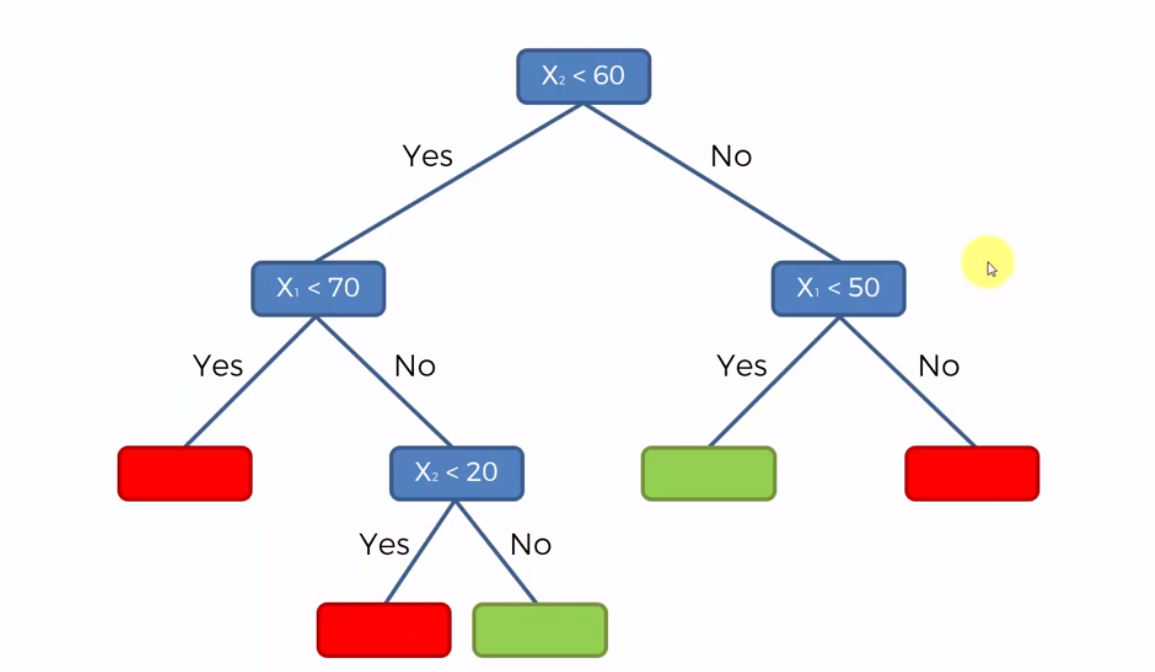CART: Classification AND Regression Trees

## Introduction

The goal of the Decision Tree Regression Algorithm is to split data into similar groups. The algorithm uses something called information entropy,which is a mathematical process (that is super complicated). The point of the algorithm is to split the data in such a way that information is added and stops splitting the data when it’s unable to add any more information to the dataset.

In the Regression section of these docs we saw the ‘Decision Tree Regression’. Remember Classification trees, classify the data and predict categories and Regression Trees predict data of real numbers. Here in this part we will be looking at how Decision Tree’s can be used to classify data but the approach is almost identical to that of the Decision Tree Regression.Here we see a graph with splits across different categories using the Decision Tree Algorithm. The decision tree makes splits in a way that would maximize the number of points in each class and tries to minimize entropy. So in plain english, it groups as many of the green and red dots together.

The decision tree would like this:This is the method that will be used to add a new data point and classify it to the graph. Additionally, we don’t always have to go all the way to the very end of the tree. Most of the time the tree will be very long and so at a resonable point you could stop and have it run a probability of where to classify the point.

For example, let’s say we stop going through the graph at X1 < 70. If the statement is True then we would have, in this case a 100% chance of it being red. Otherwise, instead goin further down the list, i could say that since there are more Green dots than red ones, I could make a prediction and say that the point will be green. This is extremly useful, as it is a lot less computationally expensive.

Decision Tree’s on their own is not a very powerful tool. However, with the help of other tools like Random Forest Trees and Gradient Boosting it has become much more powerful in the past few years.

## Decision Tree in Python (classification part)

from sklearn.tree import DecisionTreeClassifier
classifier = DecisionTreeClassifier(criterion = 'entropy', random_sate = 0)
classifier.fit(X_train, y_train)